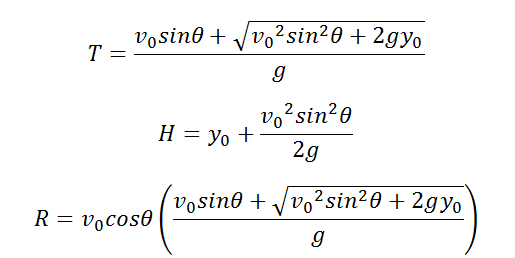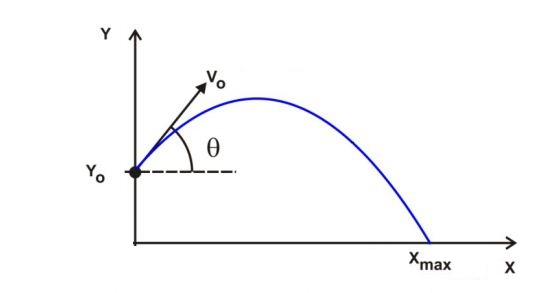# Parameters of a Parabolic Projectile from an elevation Calculator

This CalcTown calculator calculates the parameters (i.e time of flight, range and maximum height) of a point object projected from an elevation above the ground.

m
m/s

#### Result

s
m
mClick here to view image

where

T=Time of Flight

R=Range of trajectory

H=Maximum Height reached by the point object

θ=Angle made by the projectile with the horizontal

y0=Initial height from which the projectile was launched